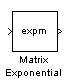# Matrix Exponential

Compute matrix exponential

## Library

Math Functions / Matrices and Linear Algebra / Matrix Operations

`dspmtrx3`

•## Description

The Matrix Exponential block computes the matrix exponential using a scaling and squaring algorithm with a Pade approximation. The input matrix must be square.

## Supported Data Types

• Double-precision floating point

• Single-precision floating point

 Array-Vector Multiply DSP System Toolbox `expm` MATLAB Dot Product Simulink Matrix Product DSP System Toolbox Product Simulink